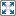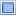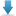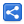# TypeScript - Type Assertions

[Last Updated: Sep 6, 2018]

In TypeScript, type assertion is a way to tell the compiler what is the type of a variable.

Type assertion is used when the type of the target variable might not be known or the programmer knows better what is the actual type of it.

Type assertion is like type casting in other languages, but in TypeScript it is only a compile time feature.

## Type assertion syntaxes

### Angle-bracket syntax

```let x: any = "hi there";
let s = (<string>x).substring(0,3);
console.log(s);

```

`hi `

### Using keyword 'as'

```let x: any = "hi there";
let s = (x as string).substring(0,3);
console.log(s);```

#### Output

`hi `

In both cases the compiled JavaScript is:

```let x = "hi there";
let s = x.substring(0, 3);
console.log(s);
```

## Example Project

Dependencies and Technologies Used:

• TypeScript 3.0.1
 TypeScript - Type AssertionsSelect AllDownload• typescript-type-assertions
• example1.ts

 Share